Function Repository Resource:

# CurvedSpacetimeRegionSprinkling

Produce random causal graphs by sprinkling points into a specified region of a (potentially curved) spacetime

Contributed by: Jonathan Gorard
 ResourceFunction["CurvedSpacetimeRegionSprinkling"][cond,{x1,…,xm},n] produces a random sprinkling of n points into a (potentially curved) spacetime region that satisfies the conditions cond. ResourceFunction["CurvedSpacetimeRegionSprinkling"][cond,{x1,…,xm},{{xmin,xmax},…},n] produces a random sprinkling of n points into a (potentially curved) spacetime region that satisfies the conditions cond restricted to the bounds {xmin,xmax}×⋯. ResourceFunction["CurvedSpacetimeRegionSprinkling"][cond,{x1,…,xm},n,"prop"] gives the property "prop" for the specified (potentially curved) spacetime region sprinkling. ResourceFunction["CurvedSpacetimeRegionSprinkling"][cond,{x1,…,xm},{{xmin,xmax},…},n,"prop"] gives the property "prop" for the specified (potentially curved) restricted spacetime region sprinkling.

## Details and Options

When no "prop" argument is given, ResourceFunction["CurvedSpacetimeRegionSprinkling"] returns a SpacetimeSprinkling object by default.
By default, the region is restricted to the bounds {{-1,1},}.
Unlike the resource function FlatSpacetimeSprinkling, sprinklings can only be performed in 0+1-, 1+1- and 2+1-dimensional spacetimes using ResourceFunction["CurvedSpacetimeRegionSprinkling"].
Sprinklings are performed using a Poisson point process, with the expected number of sprinkled points being proportional to the spacetime region volume so as to guarantee Lorentz invariance.
Causal edges are added in accordance with the standard causal partial order relation on spacetime (as determined using the usual form of the spacetime line element) and are transitively reduced by default.
ResourceFunction["CurvedSpacetimeRegionSprinkling"][cond,{x1,,xm},] treats the last variable, xm, as being the time coordinate, with {x1,,xm1} being the spatial coordinates.
ResourceFunction["CurvedSpacetimeRegionSprinkling"] uses a similar argument specification to ImplicitRegion and DiscretizeRegion.
The following properties can be requested using the "prop" argument:
 "CausalGraphFull" full causal partial order graph (before transitive reduction) "CausalGraph" transitively reduced causal partial order graph "Points" plot of the sprinkled points only (without causal edges) "PointsList" list of the spacetime coordinates of all sprinkled points "PointsCount" number of sprinkled points "Dimensions" number of dimensions in the continuum spacetime approximation "TotalEdgeCount" total number of causal edges (before transitive reduction) "ReducedEdgeCount" number of transitively reduced causal edges "PureCausalGraphFull" full causal partial order graph (before transitive reduction) with vertex coordinate information removed "PureCausalGraph" transitively reduced causal partial order graph with vertex coordinate information removed "Properties" list of properties
The sprinkling of elements of a discrete causal partial order into a continuous Lorentzian manifold via a Poisson point process was first described in the context of causal set theory by Sorkin, Bombelli and Henson.

## Examples

### Basic Examples (4)

Produce a random sprinkling of 100 points into a 1+1-dimensional hyperbolic region of spacetime:

 In:=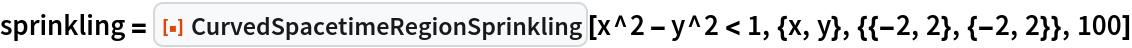Out=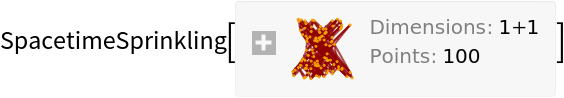Show the causal graph:

 In:=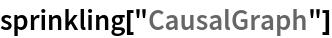Out=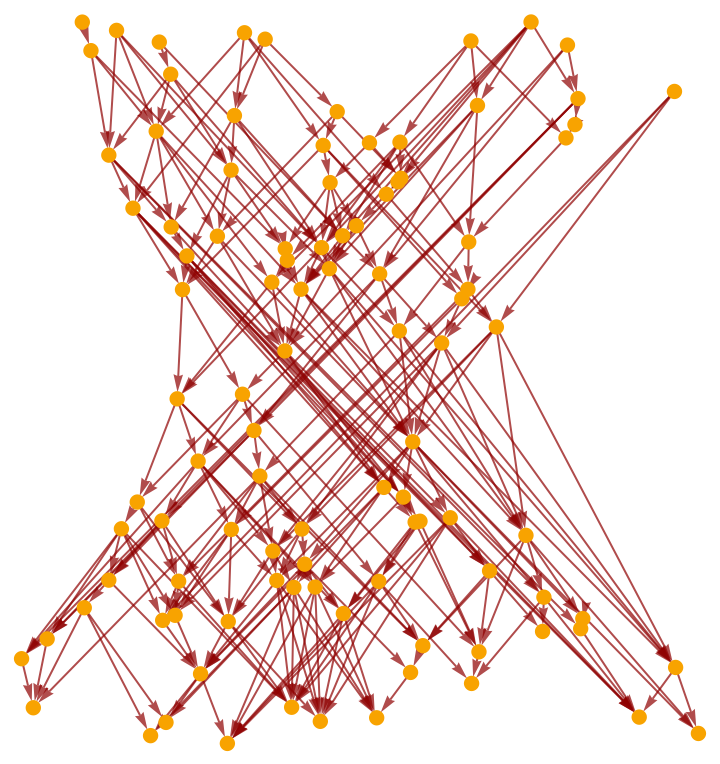Show the full causal graph (without transitive reduction):

 In:=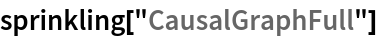Out=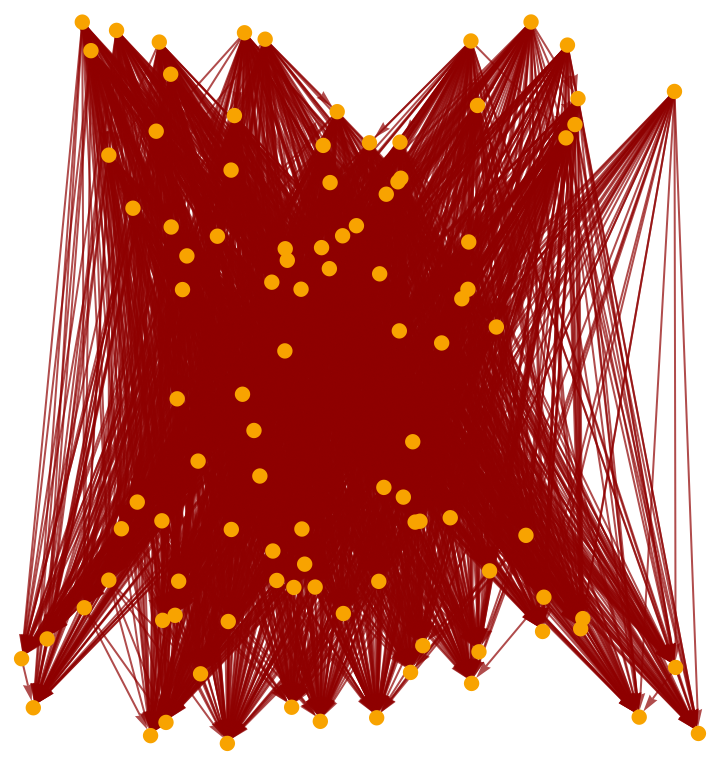Produce a random sprinkling of 200 points into a 2+1-dimensional hyperboloidal region of spacetime (i.e. de Sitter space):

 In:=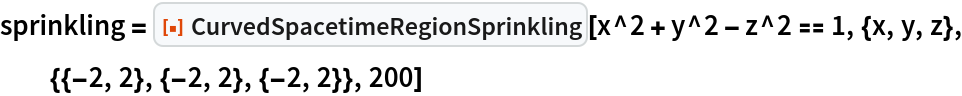Out=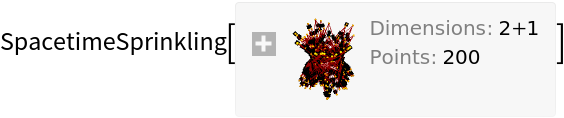Show the causal graph:

 In:=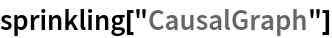Out=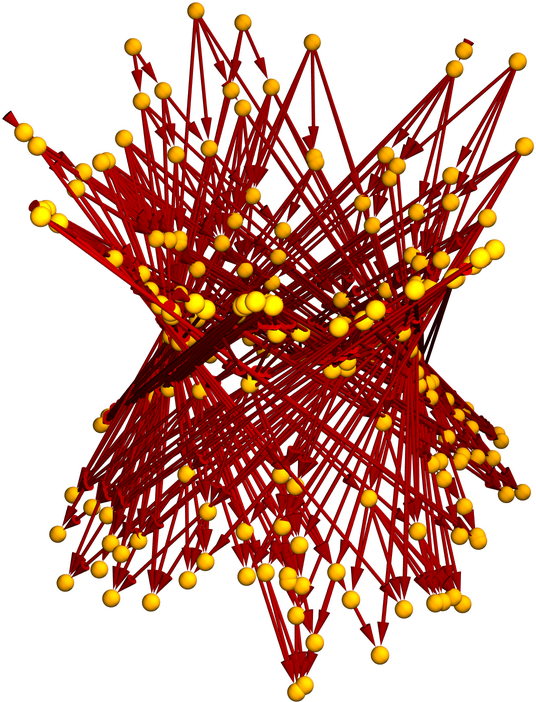Show the pure causal graph (with vertex coordinate information removed):

 In:=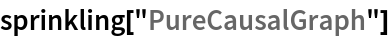Out=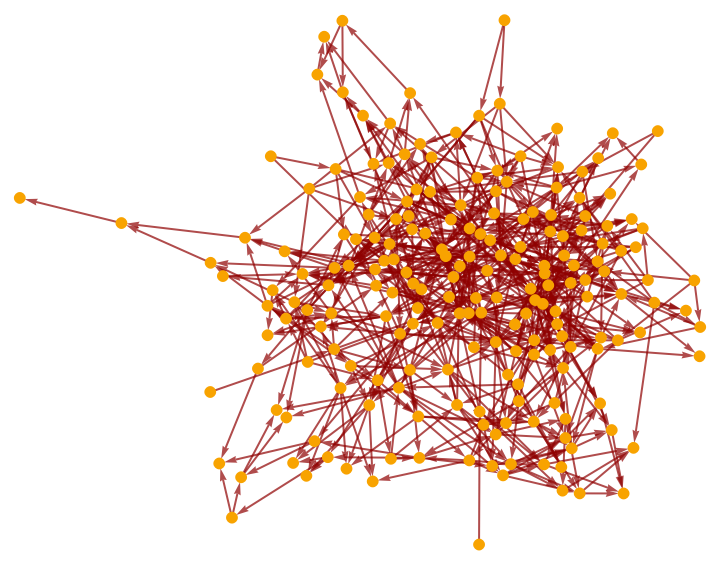Show the pure causal graph with a layered graph embedding:

 In:=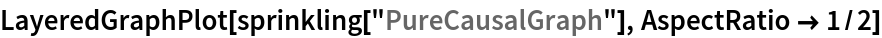Out=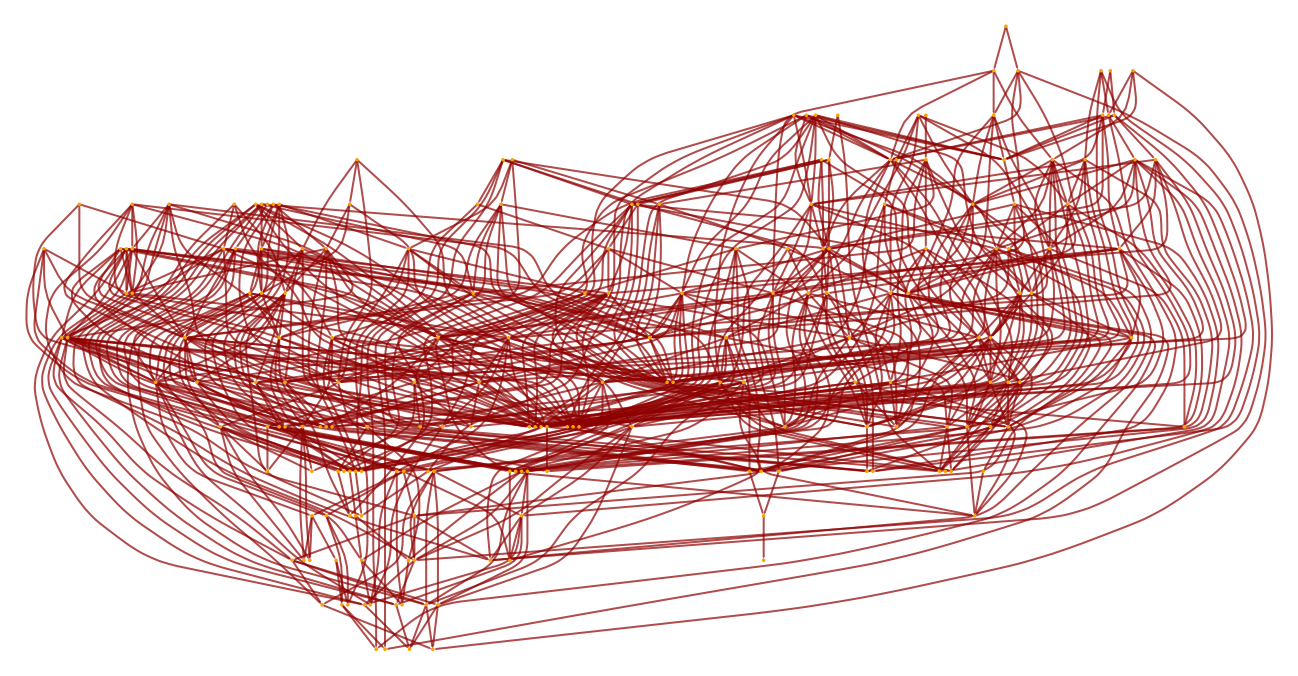Produce a random sprinkling of 200 points into a 2+1-dimensional paraboloidal region of spacetime based on Flamm's paraboloid (related to the Schwarzschild geometry):

 In:=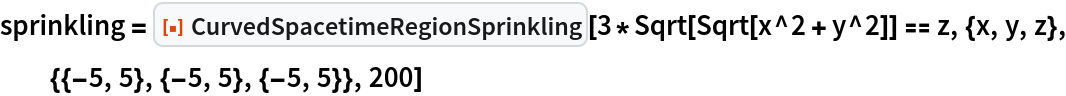Out=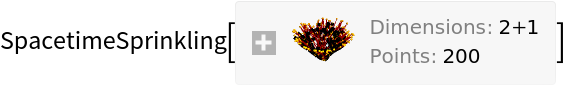Plot the positions of the sprinkled points only (without causal edges):

 In:=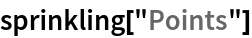Out=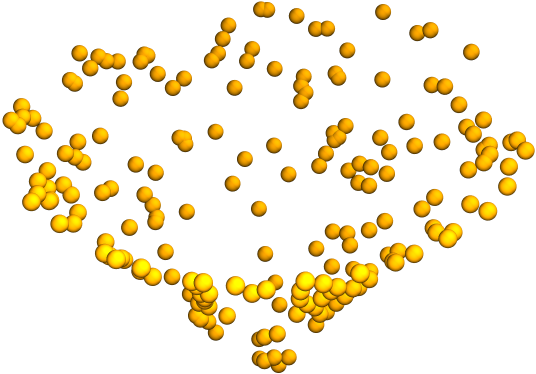Show the causal graph:

 In:=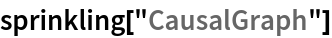Out=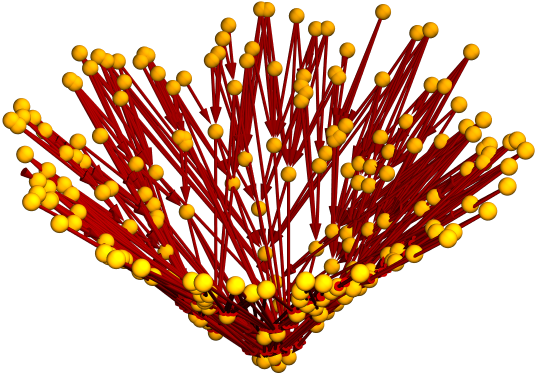By default, the region is restricted to the bounds {{-1,1},}:

 In:=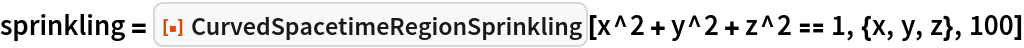Out=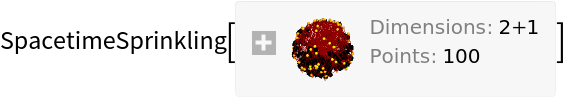Show the causal graph:

 In:=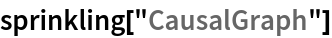Out=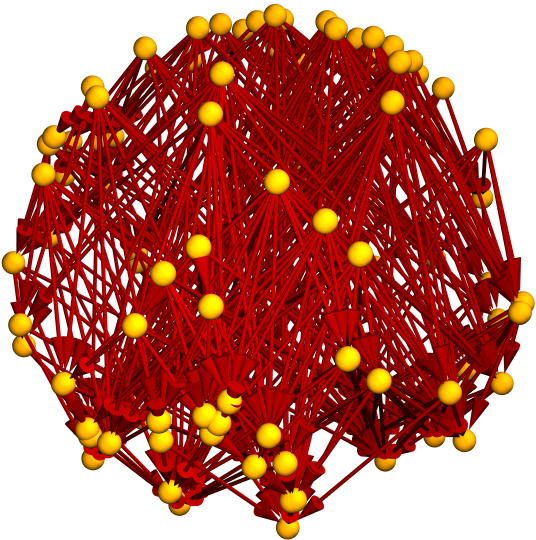### Scope (5)

Sprinklings can be produced in 0+1-dimensional spacetimes:

 In:=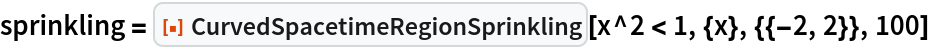Out=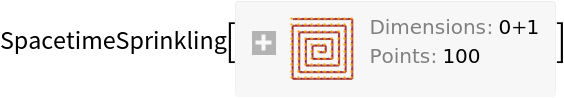Show the pure causal graph:

 In:=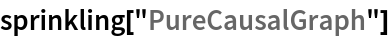Out=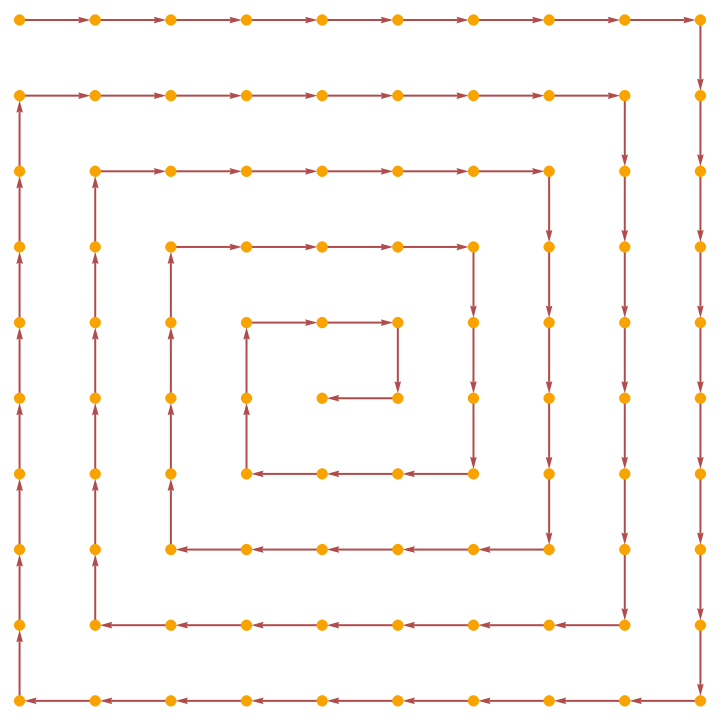Produce a sprinkling in 1+1-dimensional spacetime:

 In:=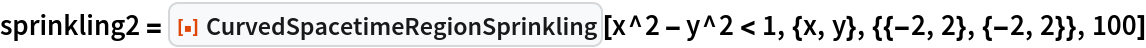Out=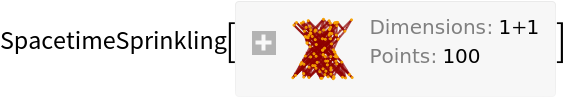Show the causal graph:

 In:=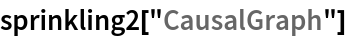Out=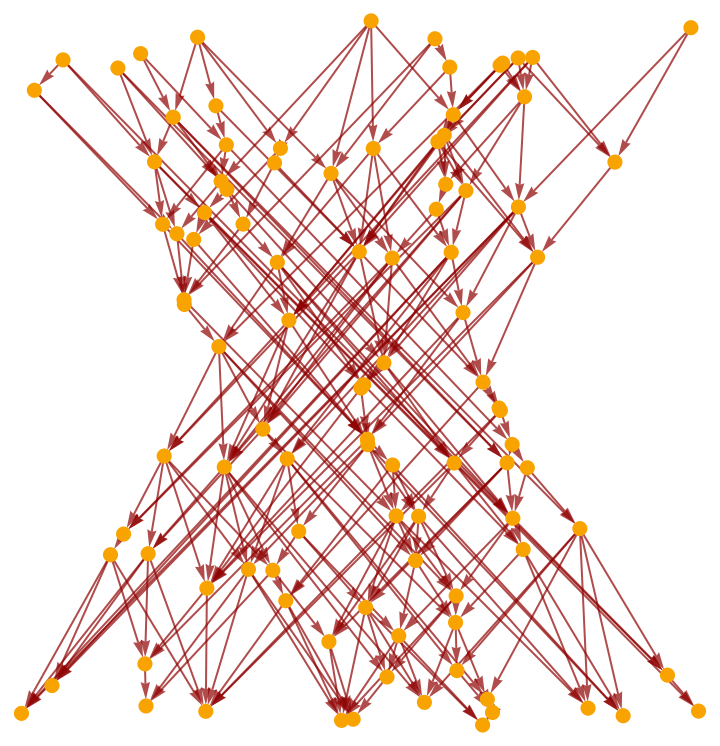Produce a sprinkling in a 2+1-dimensional spacetime:

 In:=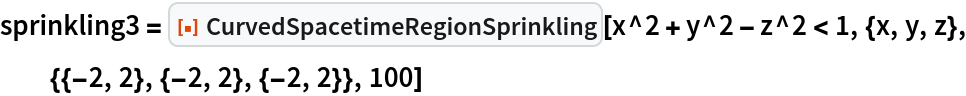Out=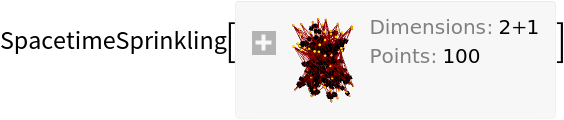Show the causal graph. Note that, unlike the resource function CurvedSpacetimeRegionSprinkling does not support higher-dimensional sprinklings:

 In:=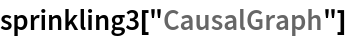Out=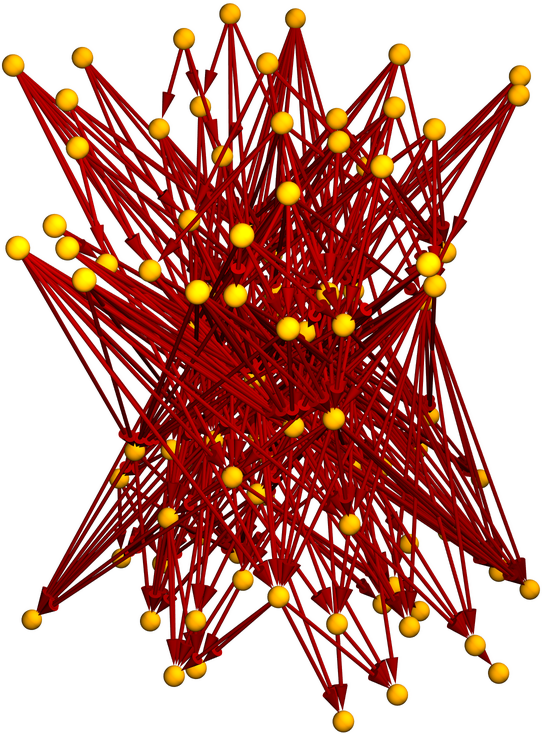Properties can be requested directly from CurvedSpacetimeRegionSprinkling:

 In:=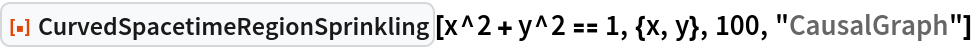Out=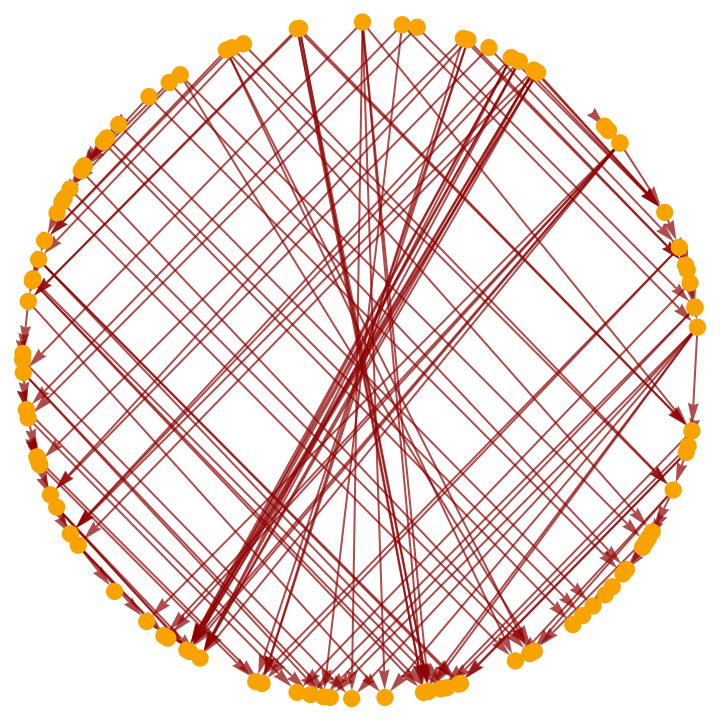Produce a random sprinkling of 100 points into a 2+1-dimensional hyperboloidal region of spacetime (i.e. de Sitter space):

 In:=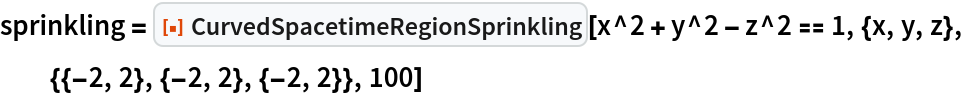Out=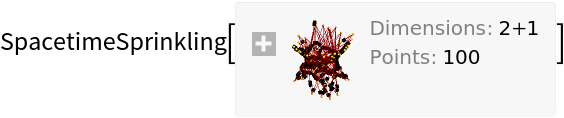Show the complete list of properties:

 In:=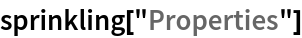Out=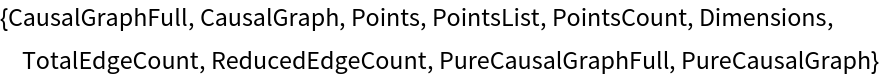Show the full causal graph:

 In:=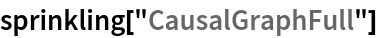Out=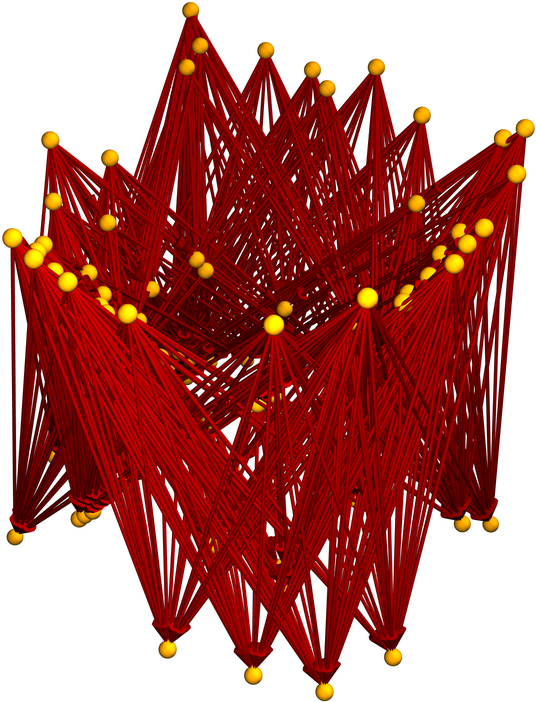Show the transitively reduced causal graph:

 In:=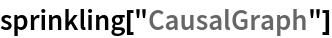Out=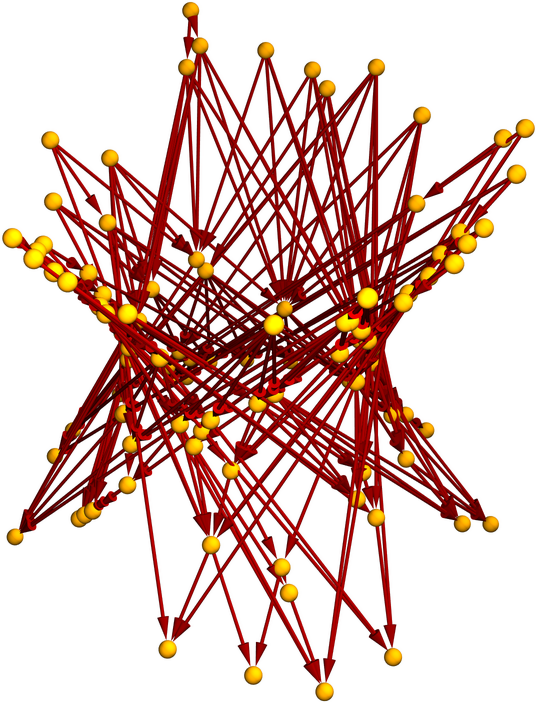Plot the positions of the sprinkled points only (without causal edges):

 In:=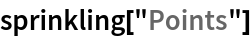Out=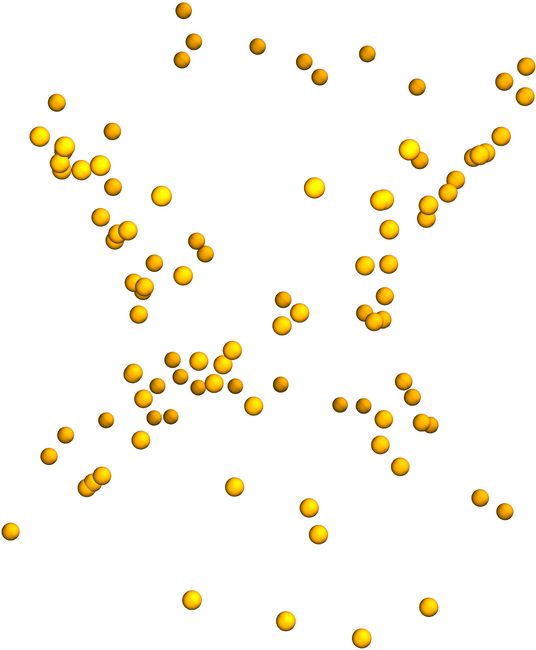Show a complete list of spacetime coordinates for the sprinkled points:

 In:=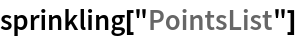Out=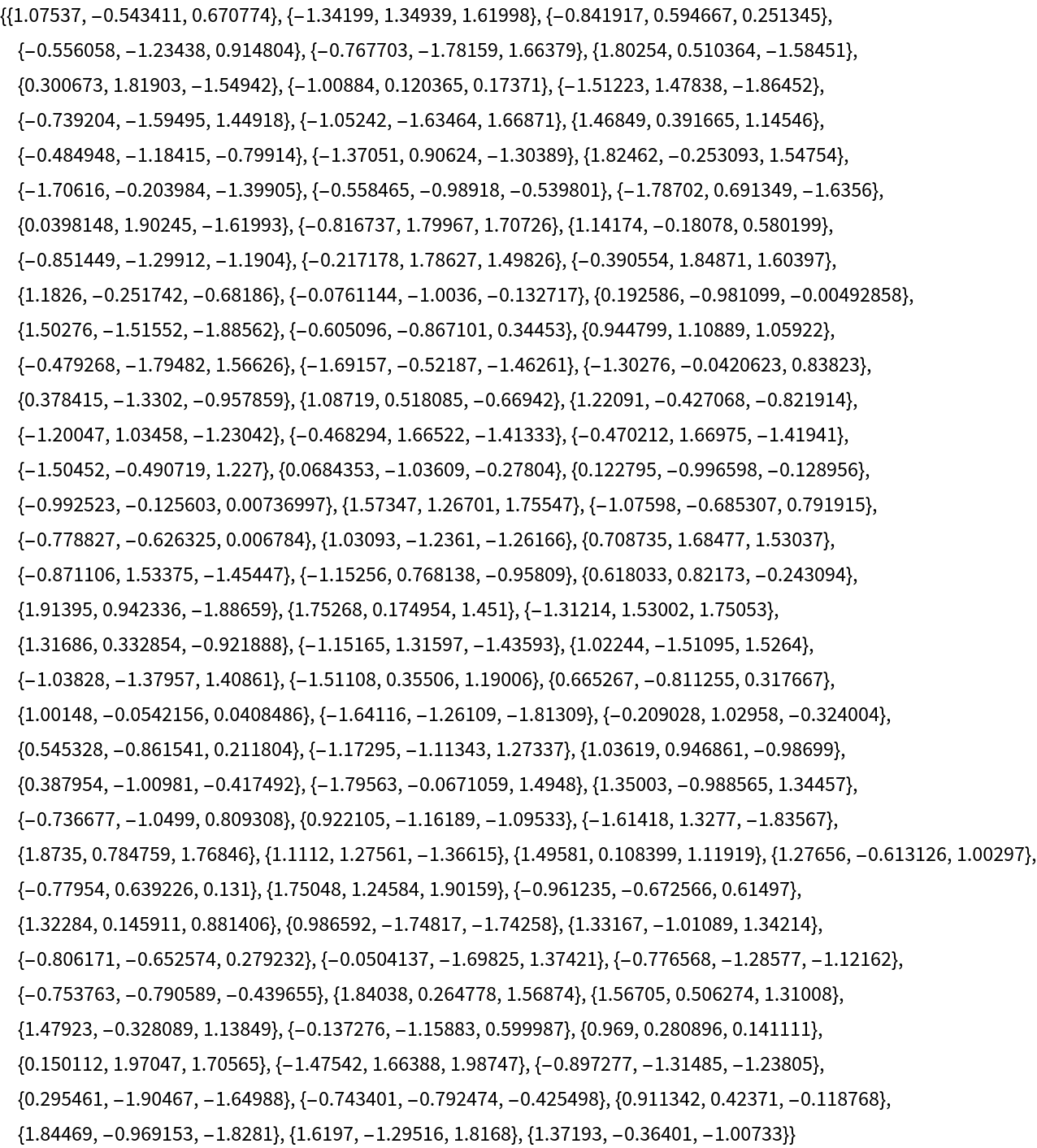Show the number of sprinkled points:

 In:=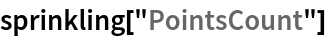Out=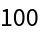Show the number of dimensions in the background spacetime:

 In:=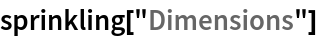Out=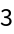Show the total number of causal edges:

 In:=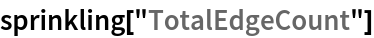Out=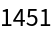Show the number of causal edges after transitive reduction:

 In:=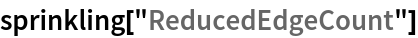Out=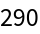Show the pure full causal graph (with vertex coordinate information removed):

 In:=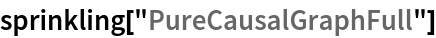Out=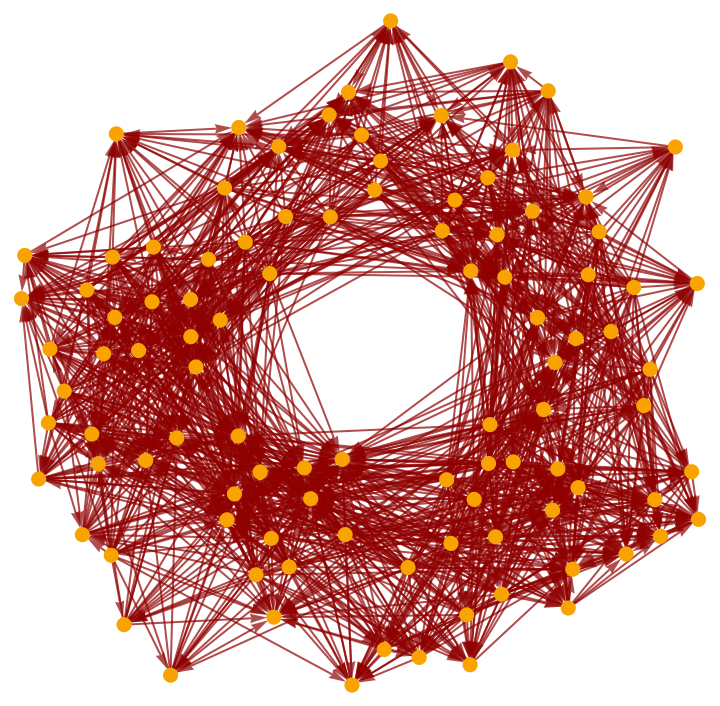Show the pure transitively reduced causal graph (with vertex coordinate information removed):

 In:=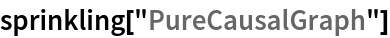Out=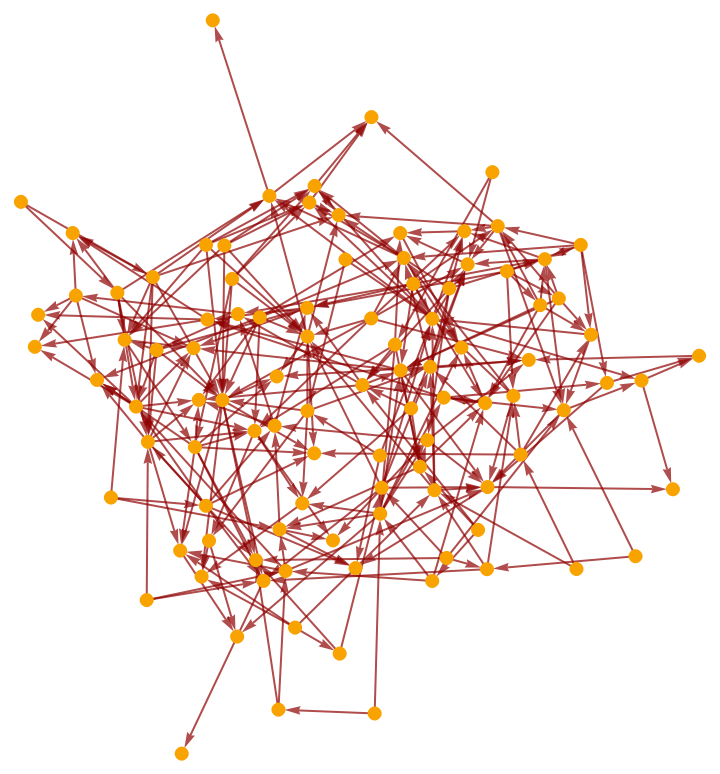Jonathan Gorard

## Version History

• 1.0.0 – 17 November 2020